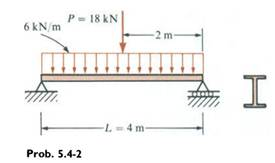### Create an Account

Home / Questions / A simple beam of length L 4 m carries a uniform load of intensity q 6 kNm acting through...

# A simple beam of length L 4 m carries a uniform load of intensity q 6 kNm acting throughout the length of the beam and a concentrated load P 18 kN acting at the midpoint see figure Assuming

A simple beam of length L = 4 m carries a uniform load of intensity q = 6 kN/m acting throughout the length of the beam and a concentrated load P = 18 kN acting at the midpoint (see figure). Assuming σallow = 124 MPa, calculate the required section modulus S. Select a suitable Universal Beam section from Table E-l, Appendix E, and recalculate S taking into account the weight of the beam. Select a new beam size if necessary.Jun 29 2020 View more View LessSubscribe To Get Solution